# Car Physics for Games

Mirror of original article by Marco Monster
Abstract: An introduction to car physics modelling for games.

version 1.9
November, 2003

#### Introduction

This tutorial is about simulating cars in games, in other words vehicle physics.

One of the key points in simplifying vehicle physics is to handle the longtitudinal and lateral forces separately.  Longtitudinal forces operate in the direction of the car body (or in the exact opposite direction).  These are wheel force, braking force, rolling resistance and drag (= airresistance).  Together these forces control the acceleration or deceleration of the car and therefore the speed of the car.  Lateral forces allow the car to turn.  These forces are caused by sideways friction on the wheels.  We'll also have a look at the angular moment of the car and the torque caused by lateral forces.

#### Notation and conventions

Vectors are shown in bold, we'll be using 2d vectors.  So the notation a = -b would translate to the following code snippet:
a.x = -b.x
a.y = -b.y

Throughout this tutorial I'll be assuming that the rear wheels provide all the drive (for four wheel drives apply the neccesary adaptations).

I'll be mainly using S.I. units (meters, kilograms, Newtons, etc.), but I've included a handy conversion table at the end for those readers more familiar with imperial measures (pounds, feet, miles, etc.)

#### Straight line physics

First let's consider a car driving in a straight line.  Which forces are at play here?  First of all there's what the tractive force, i.e. the force delivered by the engine via the rear wheels.  The engine turns the wheels forward (actually it applies a torque on the wheel), the wheels push backwards on the road surface and, in reaction, the road surface pushes back in a forward direction.  For now, we'll just say that the tractive force is equivalent in magnitude to the variable Engineforce, which is controlled directly by the user.

Ftraction = u * Engineforce,
where u is a unit vector in the direction of the car's heading.

If this were the only force, the car would accelerate to infinite speeds.  Clearly, this is not the case in real life.  Enter the resistance forces.  The first and usually the most important one is air resistance, a.k.a. aerodynamic drag.  This force is so important because it is proportional to the square of the velocity. When we're driving fast (and which game doesn't involve high speeds?) this becomes the most important resistance force.

Fdrag = - Cdrag * v * |v|
where Cdrag is a constant and v is the velocity vector and the notation |v| refers to the magnitude of vector v

The magnitude of the velocity vector is more commonly known as the speed. Note the difference of data type: speed is a scalar, velocity is a vector. Use something like the following code:

speed = sqrt(v.x*v.x + v.y*v.y);
fdrag.x = - Cdrag * v.x * speed;
fdrag.y = - Cdrag * v.y * speed;

Then there is the rolling resistance. This is caused by friction between the rubber and road surface as the wheels roll along and friction in the axles, etc.etc.. We'll approximate this with a force that's proportional to the velocity using another constant.

Frr = - Crr * v
where Crr is a constant and v is the velocity vector.

At low speeds the rolling resistance is the main resistance force, at high speeds the drag takes over in magnitude. At approx. 100 km/h (60 mph, 30 m/s) they are equal ([Zuvich]). This means Crr must be approximately 30 times the value of Cdrag

The total longtitudinal force is the vector sum of these three forces.

Flong =   Ftraction + Fdrag   + Frr

Note that if you're driving in a straight line the drag and rolling resistance forces will be in the opposite direction from the traction force.  So in terms of  magnitude, you're subtracting the resistance force from the traction force.  When the car is cruising at a constant speed the forces are in equilibrium and Flong is zero.

The acceleration (a) of the car (in meters per second squared) is determined by the net force on the car (in Newton) and the car's mass M (in kilogram) via Newton's second law:

a = F / M

The car's velocity (in meters per second) is determined by integrating the acceleration over time.  This sounds more complicated than it is, usually the following equation does the trick.  This is known as the Euler method for numerical integration.

v = v + dt * a,
where dt is the time increment in seconds between subsequent calls of the physics engine.

The car's position is in turn determined by integrating the velocity over time:

p = p + dt * v

With these three forces we can simulate car acceleration fairly accurately.  Together they also determine the top speed of the car for a given engine power. There is no need to put a maximum speed anywhere in the code, it's just something that follows from the equations.  This is because the equations form a kind of negative feedback loop.  If the traction force exceeds all other forces, the car accelerates.  This means the velocity increases which causes the resistance forces to increase.  The net force decreases and therefore the acceleration decreases.  At some point the resistance forces and the engine force cancel each other out and the car has reached its top speed for that engine power.In this diagram the X-axis denotes car velocity in meters per second and force values are set out along the Y-axis.  The traction force (dark blue) is set at an arbitrary value, it does not depend on the car velocity.  The rolling resistance (purple line) is a linear function of velocity and the drag (yellow curve) is a quadratic function of velocity.  At low speed the rolling resistance exceeds the drag.  At 30 m/s these two functions cross.  At higher speeds the drag is the larger resistance force.  The sum of the two resistance forces is shown as a light blue curve.  At 37 m/s this curve crosses the horizontal traction force line.  This is the top speed for this particular value of the engine power (37 m/s = 133 km/h = 83 mph).

#### Magic Constants

So far, we've introduced two magic constants in our equations: Cdrag and Crr .  If you're not too concerned about realism you can give these any value that looks and feels good in your game.  For example, in an arcade racer you may want to have a car that accelerates faster than any car in real life.  On the other hand, if you're really serious about realistic simulation, you'll want to get these constants exactly right.

Air resistance is approximated by the following formula (Fluid Mechanics by Landau and Lifshitz, [Beckham] chapter 6, [Zuvich])

Fdrag =  0.5 * Cd * A * rho * v2

where Cd = coefficient of friction
A is frontal area of car
rho (Greek symbol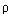)= density of air
v = speed of the car

Air density (rho) is 1.29 kg/m3 (0.0801 lb-mass/ft3), frontal area is approx. 2.2 m2 (20 sq. feet), Cd depends on the shape of the car and determined via wind tunnel tests.  Approximate value for a Corvette: 0.30.  This gives us a value for Cdrag:
Cdrag = 0.5 * 0.30 * 2.2 * 1.29
= 0.4257

We've already found that Crr should be approx. 30 times Cdrag.  This gives us
Crr = 30 * 0.4257
= 12.8

To be honest, I have my doubts about this last constant. I couldn't confirm its value anywhere. Be prepared to finetune this one to get realistic behaviour.

#### Braking

When braking, the traction force is replaced by a braking force which is oriented in the opposite direction.  The total longtitudinal force is then the vector sum of these three forces.

Flong =   Fbraking + Fdrag   + Frr

A simple model of braking:

Fbraking = -u * Cbraking

In this model the braking force is a constant.  Keep in mind to stop applying the braking force as soon as the speed is reduced to zero otherwise the car will end up going in reverse.

#### Weight Transfer

An important effect when accelerating or braking is the effect of dynamic weight transfer.  When braking hard the car will nosedive.  During accelerating, the car leans back.  This is because just like the driver is pushed back in his seat when the pedal hits the metal, so is the car's centre of mass. The effect of this is that the weight on the rear wheels increases during acceleration and the front wheels conversely have less weight to bear.

The effect of weight transfer is important for driving games for two reasons.  First of all the visual effect of the car pitching in response to driver actions adds a lot of realism to the game. Suddenly, the simulation becomes a lot more lifelike in the user's experience.

Second of all, the weight distribution dramatically affects the maximum traction force per wheel. This is because there is a friction limit for a wheel that is proportional to the load on that wheel:

Fmax = mu * W
where mu is the friction coefficient of the tyre. For street tyres this may be 1.0, for racing car tyres this can get as high as 1.5.

For a stationary vehicle the total weight of the car (W, which equals M *g) is distributed over the front and rear wheels according to the distance of the rear and front axle to the CM (c and b respectively):
Wf = (c/L)*W
Wr = (b/L)*W
where b is the distance from CG to front axle, c the distance from CG to rear axle and L is the wheelbase.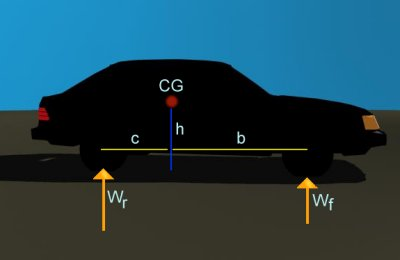If the car is accelerating or decelerating at rate a, the weight on front (Wf) and rear axle (Wr) can be calculated as follows:
Wf = (c/L)*W - (h/L)*M*a
Wr = (b/L)*W + (h/L)*M*a,
where h is the height of the CG, M is the car's mass and a is the acceleration (negative in case of deceleration).

Note that if the CG is further to the rear (c < b), then more weight falls on the rear axle and vice versa. Makes sense, doesn't it?

If you want to simplify this, you could assume that the static weight distribution is 50-50 over the front and rear axle. In other words, assume b = c = L/2. In that case, Wf = 0.5*W - (h/L)*M*a and Wr = 0.5*W +(h/L)*M*a;

#### Engine Force

When I said earlier that the engine delivers a certain amount of force, this was a a bit of a simplification.  An engine delivers an amount of torque.  Torque is like a rotational equivalent of force. Torque is force times distance. If you apply a 10 Newton force at 0.3 meters of the axis of rotation, you've got a torque of 10 x 0.3 = 3 N.m (Newton meter). That's the same torque as when you apply a 1 N force at 3 m from the axis. In both cases the leverage is the same.

The torque that an engine can deliver depends on the speed at which the engine is turning, commonly expressed as rpm (revolutions per minute). The relationship torque versus rpm is not a linear relationship, but is usually provided as a curve known as a torque curve (the exact shape and height of the curve is specific for each engine type, it is determined by engine tests). Here's an example for the 5.7 liter V8 engine found in Corvettes from 1997 to 2000: the LS1Note that the torque curve peaks at about 4400 rpm with a torque of 350 lb-ft (475 N.m) and horsepower peaks at 5600 rpm at 345 hp (257 kW). The curves are only defined in the range from, in this particular case, about 1000 to 6000 rpm, because that is the operating range of the engine. Any lower, and the engine will stall.  Any higher (above the so-called "redline"), and you'll damage it.

Oh, and by the way, this is the maximum torque the engine can deliver at a given rpm. The actual torque that the engine delivers depends on your throttle position and is a fraction between  0 and 1 of this maximum.

We're mostly interested in the torque curve, but some people find the power curve also interesting. You can derive the horsepower from the torque in foot-pounds using the following equation:

hp = torque * rpm / 5252

Because of this relationship, the two curves will always cross at 5252 rpm. Check for yourself in the diagram above.

And here's the same curves in SI units: Newton meter for torque and kiloWatt for power. The curves are the same shape, but the relative scale is different (and because of that they don't cross either).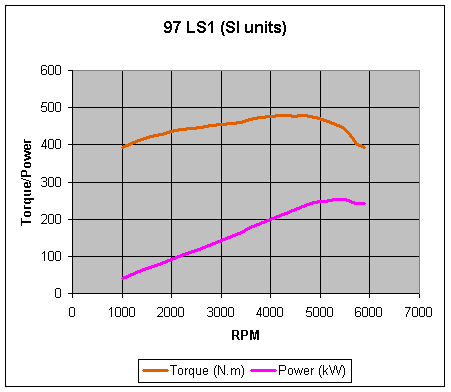Now, the torque from the engine (i.e. at the crankshaft) is converted via the gear and differential before it's applied to the rear wheels. The gearing multiplies the torque from the engine by a factor depending on the gear ratios.

Unfortunately, quite some energy is lost in the process. As much as 30% of the energy could be lost in the form of heat. This gives a so-called transmission efficiency of 70%. Let me just point out that I've seen this mentioned as a typical value, I don't have actual values for any particular car.

The torque on the rear axle can be converted to a force of the wheel on the road surface by dividing by the wheel radius. (Force is torque divided by distance).

By the way, if you want to work out the radius of a tyre from those cryptic tyre identfication codes, have a look at the The Wheel and Tyre Bible (http://www.carbibles.com ). It even provides a handy little calculator.  For example, this tells us the P275/40ZR-18 rear tyres of a Corvette have an unloaded radius of 34 cm.

Here's an equation to get from engine torque to drive force: the longtitudinal force that the two rear wheels exert on the road surface.

Fdrive = u * Tengine * xg * xd * n / Rw
where
u is a unit vector which reflects the car's orientation,
Tengine is the torque of the engine at a given rpm,
xg is the gear ratio,
xd is the differential ratio,
n is transmission efficiency and

An example:

Engine is running at 2500 rpm, looking this up on the curve gives engine torque of 448 Nm (=330 ft lbs)
Gear ratio (first gear): 2.66
Differential ratio: 3.42
Transmission efficiency: 0.7 (guess)
Wheel radius: 0.34 m (=13.4 inch)
Mass: 1500 kg (= 3300 lbs of weight) including the driver.

This gives us a potential drive force of (448*2.66*3.42*0.7/0.34 = ) 8391 N if the driver puts his foot down.

Meanwhile, in the static situation, the weight on the rear wheels is half the weight of the car and driver: (1500 kg / 2 ) * 9.8 m/s2 = 7350 N (=1650 lbs).  This means the maximum amount of traction the rear wheels can provide if mu = 1.0 is 7350 N.  Push the pedal down further than that and the wheels will start spinning and lose grip and the traction actually drops below the maximum amount.  So, for maximum acceleration the driver must exert an amount of force just below the friction threshold.  The subsequent acceleration causes a weight shift to the rear wheels.  The acceleration is:
a = 7350 N / 1500 kg  = 4.9 m/s2  (=0.5 G)

Let's say that b = c = 1.25m and L is therefore 2.50 m, the CG is 1.0 m above ground level.  After a brief moment the amount of shifted weight is then (h/L)*M*a, that is (1.0/2.50)*1500*4.9 = 2940 N.

This means Wf =  7350 - 2940 N and Wr = 7350 + 2940 N. The rear wheels now have extra weight which in this case is sufficient to allows the driver to put his foot all the way down.

#### Gear Ratios

The following gear ratios apply to an Corvette C5 hardtop (Source: http://www.idavette.net/facts/c5specs/ )

 First gear g1 2.66 Second gear g2 1.78 Third gear g3 1.3 Fourth gear g4 1 Fifth gear g5 0.74 Sixth (!) gear g6 0.5 Reverse gR 2.9 Differential ratio xd 3.42

Max torque 475 N.m (350 lb ft) at 4400 rpm, mass = 1439 kg (ignoring the driver for now). In first gear at max torque this gives us a whopping 475*2.66*3.42*0.7/0.33 =  9166 N of force. This will accelerate a mass of 1439 kg with 6.4 m/s2 (a=F/m) which equals 0.65 g.

The combination of gear and differential acts as a multiplier from the torque on the crankshaft to the torque on the rear wheels. For example, the Corvette in first gear has a multiplier of 2.66 * 3.42 = 9.1. This means each Newton meter of torque on the crankshaft results in 9.1 Nm of torque on the rear axle. Accounting for 30% loss of energy, this leaves 6.4 N.m. Divide this by the wheel radius to get the force exerted by the wheels on the road (and conversely by the road back to the wheels). Let's take a 34 cm wheel radius, that gives us 6.4 N.m/0.34 m = 2.2 N of force per N.m of engine torque. Of course, there's no such thing as a free lunch. You can't just multiply torque and not have to pay something in return. What you gain in torque, you have to pay back in angular velocity. You trade off strength for speed. For each rpm of the wheel, the engine has to do 9.1 rpm. The rotational speed of the wheel is directly related to the speed of the car (unless we're skidding). One rpm (revolution per minute) is 1/60th of a revolution per second. Each revolution takes the wheel 2 pi R further, i.e. 2 * 3.14 * 0.34 = 2.14 m. So when the engine is doing 4400 rpm in first gear, that's 483 rpm of the wheels, is 8.05 rotations per second is 17.2 m/s, about 62 km/h.

In low gears the gear ratio is high, so you get lots of torque but no so much speed. In high gears, you get more speed but less torque. You can put this in a graph as a set of curves, one for each gear, as in the following example.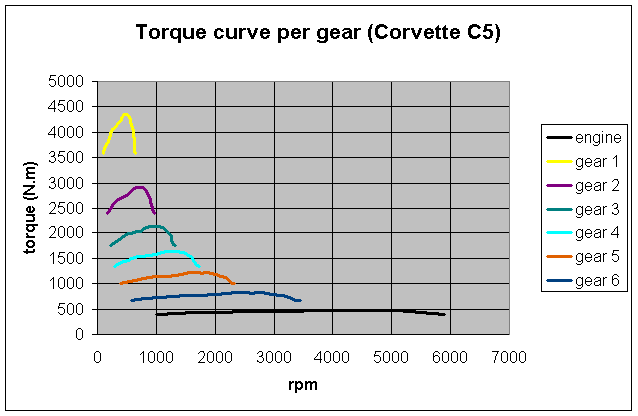Note that these curves assume a 100% efficient gearing. The engine's torque curve is shown as well for reference, it's the bottom one in black. The other curves show the torque on the rear axle for a given rpm of the axle (!), rather than the engine. As we've already seen, the rotation rate of the wheels can be related to car speed (disregarding slip for the moment) if we know the wheel radius. This means 1000 rpm of the rear axle is 36 m/s or 128 km/h car speed (80 mph). 2000 rpm is 256 km/h (160 mph). Etcetera.

#### Drive wheel acceleration

Now beware, the torque that we can look up in the torque curves above for a given rpm, is the maximum torque at that rpm. How much torque is actually applied to the drive wheels depends on the throttle position.  This position is determined by user input (the accelerator pedal) and varies from 0 to 100%. So, in pseudo-code, this looks something like this:

max torque = LookupTorqueCurve( rpm )
engine torque = throttle position * max torque

You could implement the function LookupTorqueCurve by using an array of torque/rpm value pairs and doing linear interpolation between the closest two points.

This torque is delivered to the drive wheels via the gearbox and results in what I'll call the drive torque:

drive torque = engine_torque * gear_ratio * differential_ratio * transmission_efficiency

Or written more concisely as:

Tdrive =  Tengine * xg * xd * n

Because Fdrive = Tdrive / R, this is the same as the equation for drive force we saw earlier.

Note that the gearbox increases the torque, but reduces the rate of rotation, especially in low gears.

How do we get the RPM?

So we need the rpm to calculate the engine's max torque and from there the engine's actual applied torque. In other words, now we need to know has fast the engine's crankshaft is turning.

The way I do it is to calculate this back from the drive wheel rotation speed.  After all, if the engine's not declutched, the cranckshaft and the drive wheels are physically connected through a set of gears. If we know the rpm, we can calculate the rotation speed of the drive wheels, and vice versa!

rpm = wheel rotation rate * gear ratio * differential ratio * 60 / 2 pi

The 60 / 2 pi is a conversion factor to get from rad/s to revolutions per minute.  There are 60 seconds in a minute and 2 pi radians per revolution.  According to this equation, the cranckshaft rotates faster than the drive wheels. For example, let's say the wheel is rotating at 17 rad/s.

First gear ratio is 2.66, differential ratio is 3.42 so crankshaft is rotating at 153 rad/s.
That's 153*60 = 9170 rad/minute = 9170/2 pi = 1460 rpm at the engine.

Because the torque curve isn't defined below a certain rpm, you may need to make sure the rpm is at least at some minimum value. E.g.

`if( rpm < 1000 )	rpm = 1000;`

This is needed to get the car into motion from a standstill.  The wheels aren't turning so the rpm calculation would provide zero.  At zero rpm, the engine torque is either undefined or zero, depending how your torque curve lookup is implemented. That would mean you'd never be able to get the car moving. In real life, you'd be using the clutch in this case, gently declutching while the car starts moving. So wheel rotation and engine rpm are more or less decoupled in this situation.

There are two ways to get the wheel rotation rate.  The first one is the easiest, but a bit of a quick hack.  The second one involves some more values to keep track of over time, but is more accurate and will allow for wheel spins, etcetera.

The easy way is to pretend the wheel is rolling and derive the rotation rate from the car speed and the wheel radius.

For example, let's say the car is moving at 20 km/h = 20,000 m / 3600 s = 5.6 m/s.
wheel radius is 0.33 m, so wheel angular velocity is 5.6/0.33 = 17 rad/s

Plug this into the previous equations to get the 1460 rpm, from which we can look up the engine torque on the torque curve.

The more advanced way is to let the simulation keep track of the rear wheel rotation rate and how it changes in time due to the torques that act on the rear wheels. In other words, we find the rotation rate by integrating the rotational acceleration over time.  The rotational acceleration at any particular instant depends on the sum of all the torques on the axle and equals the net torque divided by the rear axles inertia (just like linear accelaration is force divided by mass). The net torque is the drive torque we saw earlier minus the friction torques that counteract it (braking torque if you're braking and traction torque from the contact with the road surface).

#### Slip ratio and traction force

Calculating the wheel angular velocity from the car speed is only allowed if the wheel is rolling, in other words if there is no lateral slip between the tyre surface and the road.  This is true for the front wheels, but for drive wheels this is typically not true. After all, if a wheel is just rolling along it is not transfering energy to keep the car in motion.

In a typical situation where the car is cruising at constant speed, the rear wheels will be rotating slighty faster than the front wheels . The front wheels are rolling and therefore have zero slip. You can calculate their angular velocity by just dividing the car speed by 2 pi times the wheel radius.  The rear wheels however are rotating faster and that means the surface of the tyre is slipping with regard to the road surface.  This slip causes a friction force in the direction opposing the slip. The friction force will therefore be pointing to the front of the car.  In fact, this friction force, this reaction to the wheel slipping, is what pushes the car forwards.  This friction force is known as traction or as the longtitudinal force.  The traction depends on the amount of slip.  The standardised way of expressing the amount of slip is as the so-called slip ratio: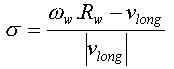wherew (pronounced: omega) is wheel angular velocity (in rad/s)
Rw is wheel radius (in m)
vlong is car speed a.k.a. longtitudinal velocity (in m/s)

Note: there are a number of slightly different definitions for slip ratio in use. This particular definition also works for cars driving in reverse.

Slip ratio is zero for a free rolling wheel.  For a car braking with locked wheels the slip ratio is -1, and a car accelerating forward has a positive slip ratio. It can even be larger than 1 of there's a lot of slip.

The relationship between longtitudinal (forward) force and slip ratio can be described by a curve such as the following: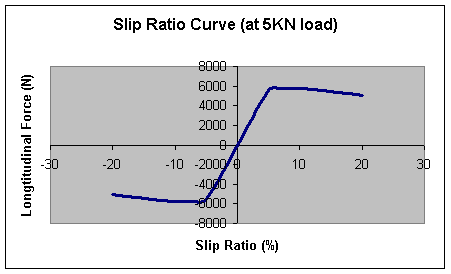Note how the force is zero if the wheel rolls, i.e. slip ratio = 0, and the force is at a peak for a slip ratio of approximately 6 % where the longtitudinal force slightly exceeds the wheel load. The exact curve shape may vary per tyre, per road surface, per temperature, etc. etc.

That means a wheel grips best with a little bit of slip. Beyond that optimum, the grip decreases. That's why a wheel spin, impressive as it may seem, doesn't actually give you the best possible acceleration.  There is so much slip that the longtitudinal force is below its peak value. Decreasing the slip would give you more traction and better acceleration.

The longtitudinal force has (as good as) a linear dependency on load, we saw that earlier when we dicussed weight transfer.  So instead of plotting a curve for each particular load value, we can just create one normalized curve by dividing the force by the load.

To get from normalized longtitudinal force to actual longtitudinal force, multiply by the load on the wheel.

Flong = Fn, long * Fz
where Fn, long is the normalized longtitudinal force for a given slip ratio and Fz is the load on the tyre.

For a simple simulation the longtitudinal force can be approximated by a linear function:

Flong = Ct * slip ratio

where Ct is known as the traction constant, which is the slope of the slip ratio curve at the origin. Cap the force to a maximum value so that the force doesn't increase after the slip ratio passes the peak value. If you plot that in a curve, you get the following: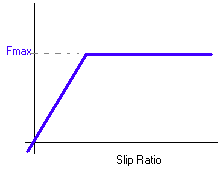#### Torque on the drive wheels

To recap, the traction force is the friction force that the road surface applies on the wheel surface.  Obviously, this force will cause a torque on the axis of each drive wheel:

traction torque = traction force * wheel radius

This torque will oppose the torque delivered by the engine to that wheel (which we called the drive torque).  If the brake is applied this will cause a torque as well. For the brake, I'll assume it delivers a constant torque in the direction opposite to the wheel' s rotation. Take care of the direction, or you won't be able to brake when you're reversing.

The following diagram illustrates this for an accelerating car.  The engine torque is magnified by the gear ratio and the differential ratio and provides the drive torque on the rear wheels.  The angular velocity of the wheel is high enough that it causes slip between the tyre surface and the road, which can be expressed as a positive slip ratio. This results in a reactive friction force, known as the traction force, which is what pushed the car forward.  The traction force also results in a traction torque on the rear wheels which opposes the drive torque.  In this case the net torque is still positive and will result in an acceleration of the rear wheel rotation rate. This will increase the rpm and increase the slip ratio.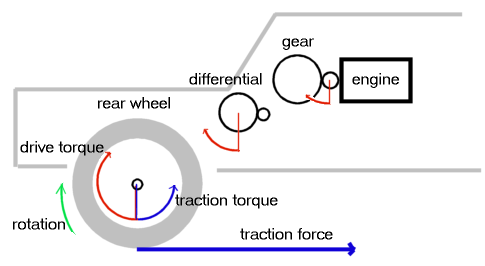The net torque on the rear axle is the sum of following torques:

total torque = drive torque + traction torques from both wheels + brake torque

Remember that torques are signed quantities, the drive torque wil generally have a different sign than the traction and brake torque. If the driver is not braking, the brake torque is zero.

This torque will cause an angular acceleration of the drive wheels, just like a force applied on a mass will cause it to accelerate.

angular acceleration = total torque / rear wheel inertia.

I've found somewhere that the inertia of a solid cylinder around its axis can be calculated as follows:

inertia of a cylinder = Mass * radius2 / 2

So for a 75 kg wheel with a 33 cm radius that's an inertia of 75 *  0.33* 0.33 / 2 = 4.1 kg.mYou must double that to get the total inertia of both wheels on the rear axle and perhaps add something for the inertia of the axle itself, the inertia of the gears and the inertia of the engine.

A positive angular acceleration will increase the angular velocity of the rear wheels over time. As for the car velocity which depends on the linear acceleration, we simulate this by doing one step of numerical integration each time we go through the physics calculation:

rear wheel angular velocity += rear wheel angular acceleration * time step

where time step is the amount of simulated time between two calls of this function.  This way we can determine how fast the drive wheels are turning and therefore we can calculate the engine's rpm.

The Chicken and the Egg

Some people get confused at this point.  We need the rpm to calculate the torque, but the rpm depends on the rear wheel rotation rate which depends on the torque. Surely, this is a circular definition, a chicken and egg situation?

This is an example of a Differential Equation: we have equations for different variables that mutually depend on each other. But we've already seen another example of this before: air resistance depends on speed, yet the speed depends on air resistance because that influences the acceleration.

To solve differential equations in computer programs we use the technique of numeric integration: if we know all the values at time t, we can work out the values at time t + delta.  In other words, rather than trying to solve these mutually dependent equations by going into an infinite loop, we take snapshots in time and plug in values from the previous snapshot to work out the values of the new snapshot.  Just use the old values from the previous iteration to calculate the new values for the current iteration.  If the time step is small enough, it will give you the results you need.

There's a lot of theory on differential equations and numeric integration.  One of the problems is that a numeric integrator can "blow up" if the time step isn't small enough.  Instead of well-behaved values, they suddenly shoot to infinity because small errors are multiplied quickly into larger and larger errors.  Decreasing the time step can help, but costs more cpu cycles. An alternative is to use a smarter integrator, e.g. RK4.  For more information on this topic, see my article on numerical stability:Achieving a Stable Simulator

Curves

Okay, enough of driving in a straight line. How about some turning?

One thing to keep in mind is that simulating the physics of turning at low speed is different from turning at high speed. At low speeds (parking lot manoeuvres), the wheels pretty much roll in the direction they're pointed at. To simulate this, you need some geometry and some kinetics. You don't really need to consider forces and mass. In other words, it is a kinetics problem not a dynamics problem.

At higher speeds, it becomes noticeable that the wheels can be heading in one direction while their movement is still in another direction. In other words, the wheels can sometimes have a velocity that is is not aligned with the wheel orientation. This means there is a velocity component that is at a right angle to the wheel. This of course causes a lot of friction. After all a wheel is designed to roll in a particular direction and that without too much effort. Pushing a wheel sideways is very difficult and causes a lot of friction force. In high speed turns, wheels are being pushed sideways and we need to take these forces into account.

Let's first look at low speed cornering.  In this situation we can assume that the wheels are moving in the direction they're pointing.  The wheels are rolling, but not slipping sideways.  If the front wheels are turned at an angle delta and the car is moving at a constant speed, then the car will describe a circular path.  Imagine lines projecting from the centre of the hubcabs of the front and rear wheel at the inside of the curve.  Where these two lines cross, that's the centre of the circle.

This is nicely illustrated in the following screenshot. Note how the green lines all intersect in one point, the centre round which the car is turning.  You may also notice that the front wheels aren't turned at the same angle, the outside wheel is turned slightly less than the inside wheel.  This is also what happens in real life, the steering mechanism of a car  is designed to turn the wheels at a different angle. For a car simulation, this subtlety is probably not so important.  I'll just concentrate on the steering angle of the front wheel at the inside of the curve and ignore the wheel at the other side.This is a screen shot from a car physics demo by Rui Martins.
http://ruimartins.net/software/projects/racer/

The radius of the circle can be determined via geometry as in the following diagram:The distance between front and rear axle is known at the wheel base and denoted as L.  The radius of the circle that the car describes (to be precise the circle that the front wheel describes) is called R.  The diagram shows a triangle with one vertex in the circle centre and one at the centre of each wheel.  The angle at the rear wheel is 90 degrees per definition.  The angle at the front wheel is 90 degrees minus delta.  This means the angle at the circle centre is also delta (the sum of the angles of a triangle is always 180 degrees).  The sine of this angle is the wheel base divided by the circle radius, therefore:Note that if the steering angle is zero, then the circle radius is infinite, i.e. we're driving in a straight line.

Okay, so we can derive the circle radius from the steering angle, now what?  Well, the next step is to calculated the angular velocity, i.e. the rate at which the car turns.  Angular velocity is usually represented using the Greek letter omega (), and is expressed in radians per second. (A radian is a full circle divided by 2 pi). It is fairly simple to determine: if we're driving circles at a constant speed v and the radius of the circle is R, how long does it take to complete one circle?  That's the circumference divided by the speed.  In the time the car has described a circular path it has also rotated around its up-axis exactly once. In other words:By using these last two equations, we know how fast the car must turn for a given steering angle at a specific speed.  For low speed cornering, that's all we need.  The steering angle is determined from user input. The car speed is determined as for the straight line cases (the velocity vector always points in the car direction). From this we calculate the circle radius and the angular velocity.  The angular velocity is used to change the car orientation at a specific rate. The car's speed is unaffected by the turn, the velocity vector just rotates to match the car's orientation.

#### High Speed Turning

Of course, there are not many games involving cars that drive around sedately (apart from the legendary Trabant Granny Racer ;-).  Gamers are an impatient lot and usually want to get somewhere in a hurry, preferably involving some squealing of tires, grinding of gearboxes and collateral damage to the surrounding environment.  Our goal is to find a physics model that will allow understeer,oversteer, skidding, handbrake turns, etc.

At high speeds, we can no longer assume that wheels are moving in the direction they're pointing.  They're attached to the car body which has a certain mass and  takes time to react to steering forces.  The car body can also have an angular velocity.  Just like linear velocity, this takes time to build up or slow down.  This is determined by angular acceleration which is in turn dependent on torque and inertia (which are the rotational equivalents of force and mass).

Also, the car itself will not always be moving in the direction it's heading.  The car may be pointing one way but moving another way.  Think of rally drivers going through a curve.  The angle between the car's orientation and the car's velocity vector is known as the sideslip angle (beta).Now let's look at high speed cornering from the wheel's point of view. In this situation we need to calculate the sideways speed of the tires. Because wheels roll, they have relatively low resistance to motion in forward or rearward direction. In the perpendicular direction, however, wheels have great resistance to motion. Try pushing a car tire sideways. This is very hard because you need to overcome the maximum static friction force to get the wheel to slip.

In high speed cornering, the tires develop lateral forces also known as the cornering force. This force depends on the slip angle (alpha), which is the angle between the tire's heading and its direction of travel. As the slip angle grows, so does the cornering force. The cornering force per tire also depends on the weight on that tire. At low slip angles, the relationship between slip angle and cornering force is linear, in other words

Flateral = Ca * alpha

where the constant C is known as the cornering stiffness.

If you'd like to see this explained in a picture, consider the following one. The velocity vector of the wheel has angle alpha relative to the direction in which the wheel can roll. We can split the velocity vector v up into two component vectors. The longtitudinal vector has magnitude cos(alpha) * v. Movement in this direction corresponds to the rolling motion of the wheel. The lateral vector has magnitude sin(alpha) * v and causes a resistance force in the opposite direction: the cornering force.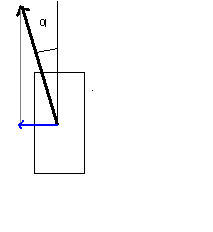There are three contributors to the slip angle of the wheels: the sideslip angle of the car, the angular rotation of the car around the up axis (yaw rate) and, for the front wheels, the steering angle.

The sideslip angle b (bèta) is the difference between the car orientation and the direction of movement. In other words, it's the angle between the longtitudinal axis and the actual direction of travel. So it's similar in concept to what the slip angle is for the tyres. Because the car may be moving in a different direction than where it's pointing at, it experiences a sideways motion. This is equivalent to the perpendicular component of the velocity vector.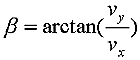If the car is turning round the centre of geometry (CG) at a rate omega (in rad/s!), this means the front wheels are describing a circular path around CG at that same rate. If the car turns full circle, the front wheel describes a circular path of distance 2.pi.b around CG in 1/(2.pi.omega) seconds where b is the distance from the front axle to the CG. This means a lateral velocity of omega * b. For the rear wheels, this is -omega * c. Note the sign reversal. To express this as an angle, take the arctangent of the lateral velocity divided by the longtitudinal velocity (just like we did for beta).  For small angles we can approximate arctan(vy/vx) by vx/vy.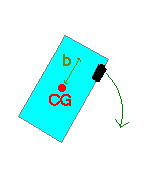The steering angle (delta) is the angle that the front wheels make relative to the orientation of the car. There is no steering angle for the rear wheels, these are always in line with the car body orientation. If the car is reversing, the effect of the steering is also reversed.The slip angles for the front and rear wheels are given by the following equations:The lateral force exercised by the tyre is a function of the slip angle.  In fact, for real tyres it's quite a complex function once again best described by curve diagrams, such as the following:What this diagram shows is how much lateral (sideways) force is created for any particular value of the slip angle.  This type of diagram is specific to a particular type of tyre, this is a fictitious but realistic example.  The peak is at about 3 degrees.  At that point the lateral force even slightly exceeds the 5KN load on the tyre.

This diagram is similar to the slip ratio curve we saw earlier, but don't confuse them. The slip ratio curve gives us the forward force depending on amount of longtitudinal slip.  This curve gives us the sideways (lateral) force depending on slip angle.

The lateral force not only depends on the slip angle, but also on the load on the tyre.  This is a plot for a load of 5000 N, i.e. the weight exerted by about 500 kg of mass pushing down on one tyre. Different curves apply to different loads because the weight changes the tyre shape and therefore its properties. But the shape of the curve is very similar apart from the scaling, so we can approximate that the lateral force is linear with load and create a normalized lateral force diagram by dividing the lateral force by the 5KN of load.

Flateral = Fn, lat * Fz
where Fn, lat is the normalized lateral force for a given slip angle and Fz is the load on the tyre.

For very small angles (below the peak) the lateral force can be approximated by a linear function:

Flateral = Ca * alpha
The constant Ca goes by the name of the cornering stiffness. This is the slope of the diagram at slip angle 0.

If you want a better approximation of the the relationship between slip angle and lateral force, search for information on the so-called Pacejka Magic Formula, names after professor Pacejka who developed this at Delft University.  That's what tyre physicists use to model tyre behaviour. It's a set of equations with lots of "magic" constants.  By choosing the right constants these equations provide a very good approximation of curves found through tyre tests.  The problem is that tyre manufactors are very secretive about the values of these constants for actual tyres. So on the one hand, it's a very accurate modeling technique. On the other hand, you'll have a hard time finding good tyre data to make any use whatsoever of that accuracy.

The lateral forces of the four tyres have two results: a net cornering force and a torque around the yaw axis.  The cornering force is the force on the CG at a right angle to the car orientation and serves as the centripetal force which is required to describe a circular path.  The contribution of the rear wheels to the cornering force is the same as the lateral force.  For the front wheels, multiply the lateral force with cos(delta) to allow for the steering angle.

Fcornering = Flat, rear + cos(delta) * Flat, front
As a point of interest, we can find the circle radius now that we know the centripetal force using the following equation
Fcentripetal = M v2 / radius
The lateral force also introduce a torque which causes the car body to turn.  After all, it would look very silly if the car is describing a circle but keeps pointing in the same direction.  The cornering force makes sure the CG describes a circle, but since it operates on a point mass it does nothing about the car orientation. That's what we need the torque around the yaw axis for.

Torque is force multiplied by the perpendicular distance between the point where the force is applied and the pivot point.  So for the rear wheels the contribution to the torque is -Flat, rear * c and for the front wheels it's cos(delta) * Flat, front * b.  Note that the sign differs.

Applying torque on the car body introduces angular acceleration.  Just like Newton's second law F = M.a, there is a law for torque and angular acceleration:

Torque = Inertia * angular acceleration.

The inertia for a rigid body is a constant which depends on its mass and geometry (and the distribution of the mass within its geometry).  Engineering handbooks provide formulas for the inertia of common shapes such as spheres, cubes, etc.

Epilogue

You can download a demo (car demo v0.8) and the source code which demonstrates high speed cornering using the method just described. Or easier yet, try the Java version by Marcel "Bloemschneif" Sodeike!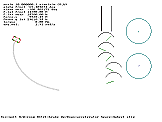The following information is shown on screen:

• steering angle (also known as delta)
• side slip angle (also known as beta)
• "rotation angle" (due to yaw rate)
• slip angle front wheels
• slip angle rear wheels
• velocity vector relative to car reference frame
• velocity vector in world spaceSee also my work in progress on the game Downtown Drivin' for an example of some of the stuff that was discussed here.

If you spot mistakes in this tutorial or find sections that could do with some clarification, let me know.

-Marco-

#### References

[Beckham] Physics of Racing Series, Brian Beckham,
http://phors.locost7.info

[Bower] Richard Bower,
http://www.dur.ac.uk/~dph0rgb

[Hecker] Chris Hecker, series on Physics in Game Development Magazine,
http://www.d6.com/users/checker/dynamics.htm

[Lander] Jeff Lander, The Trials and Tribulations of Tribology,
http://www.gamasutra.com/features/20000510/lander_01.htm

[RQRiley] Automobile Ride, Handling and Suspension Design, R.Q. Riley Enterprises,
http://www.rqriley.com/suspensn.html

[Zuvich] Vehicle Dynamics for Racing Games, Ted Zuvich,
http://www.gdconf.com/2000/library/homepage.htm, note that you need to obtain a (free) Gamasutra id to access the game development conference archives.

Car specifications

It's handy to know what sort of values to use for all these different constants we've seen.  Preferably some real values from some real cars. I've collected what I could for a Corvette C5. Be warned: some of the data is still guesswork.

#### Units and Conversions

The following S.I. units apply:

 Force N (Newton) = m.kg/s2 Power W (Watt) = N.m/s = J/s = m2 kg / s3 Torque N.m (Newton meter) Speed m/s Angular velocity rad/s Acceleration m/s2 Mass kg Distance m
Angle rad =360 degrees/ 2 pi

Here are some handy unit conversions to S.I. units.

 1 mile = 1.6093 km 1 ft (foot) = 0.3048 m 1 in (inch) = 0.0254 m = 2.54 cm 1 km/h = 0.2778 m/s 1 mph = 1.609 km/h = .447 m/s 1 rpm (revolution per minute) = 0.105 rad/s 1 G = 9.8 m/s2 = 32.1 lb/s2 1 lb (pound) = 4.45 N 1 lb (pound) = 0.4536 kg 1) = 1 lb/1G 1 lb.ft (foot pounds) = 1.356 N.m 1 lb.ft/s (foot pound per second) = 1.356 W 1 hp (horsepower) = 550 ft.lb/s = 745.7 W 1 metric hp = 0.986 hp = 735.5 W

1) To say a pound equals so and so much kilograms is actually nonsense. A pound is a unit of force and a kilogram is a unit of mass. What we mean by this "conversion" is that one pound of weight (which is a force) equals the weight exerted by 0.4536 kg of mass assuming the gravity is 9.8m/s2. On the moon, a kilogram will weigh less but still have the same mass.

Unit converter on the web: http://lecture.lite.msu.edu/~mmp/conversions/intro.htm

Marco Monster
E-mail: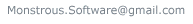Website: http://home.planet.nl/~monstrous